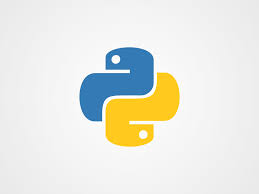## Weekend Python Project: ROCK , SCISSORS and PAPER.January 10, 2021

Rock paper scissors game is also known as stone paper scissors. It is a hand game that is usually played between 2 people, each player can randomly form any one of three from their hand. A player who chooses rock will win by another player who chooses scissors but loose by the player who chooses paper; a player with paper will loose by the player with the scissors. If both players choose the same then the game is tied. Rock paper scissors game is mainly played among kids.

Source Code

``````import random

# Game Rules
print("******************************")
print("GAME RULES :\n"+"Rock vs Paper->Paper Wins \n"+"Rock vs Scissor->Rock Wins \n"+"Paper vs Scissor->Scissor Wins")
print("*******************************")
while True:
print("Enter choice \n 1. Rock \n 2. Paper \n 3. Scissor")

# User Turn : Take the input from User
user_choice = int(input("User turn: "))

# looping until user enter invalid input
while user_choice > 3 or user_choice < 1:
user_choice = int(input("Invalid Input !!! Enter Valid input: "))

# Assigne the value to  choice_name variable
# corresponding to the choice value
if user_choice == 1:
choice_name = 'Rock'
elif user_choice == 2:
choice_name = 'Paper'
else:
choice_name = 'Scissor'
# print user choice

print("User Choice is: " + choice_name)

print("\n Now its computer turn.......")

# Computer chooses randomly any number
# among 1 , 2 and 3. Using randint method
# of random module
computer_choice = random.randint(1, 3)

# looping until comp_choice value
# is equal to the choice value
while computer_choice == choice:
computer_choice = random.randint(1, 3)

# initialize value of comp_choice_name
# variable corresponding to the choice value
if computer_choice == 1:
computer_choice_name = 'Rock'
elif computer_choice == 2:
computer_choice_name = 'Paper'
else:
computer_choice_name = 'scissor'

print("Computer choice is: " + computer_choice_name)

print(choice_name + " V/s " + computer_choice_name)

# condition for winning
if((choice == 1 and computer_choice == 2) or
(choice == 2 and computer_choice ==1 )):
print("Paper wins => ", end = "")
result = "Paper"

elif((choice == 1 and computer_choice == 3) or
(choice == 3 and computer_choice == 1)):
print("Rock Wins =>", end = "")
result = "Rock"
else:
print("Scissor Wins =>", end = "")
result = "Scissor"

# Printing either user or computer wins
if result == choice_name:
print("<== User wins ==>")
else:
print("<== Computer wins ==>")

print("Do you want to play again? (Y/N)")
ans = input()

# if user input n or N then condition is True
if ans == 'n' or ans == 'N':
break

# after coming out of the while loop
# we print thanks for playing
print("\n Thanks for playing")
``````

Output :

```******************************
GAME RULES :
Rock vs Paper->Paper Wins
Rock vs Scissor->Rock Wins
Paper vs Scissor->Scissor Wins
*******************************
Enter choice
1. Rock
2. Paper
3. Scissor
User turn: 2
User Choice is: Paper

Now its computer turn.......
Computer choice is: Paper
Paper V/s Paper
Paper wins => <== User wins ==>
Do you want to play again? (Y/N)
y
Enter choice
1. Rock
2. Paper
3. Scissor
User turn: 3
User Choice is: Scissor

Now its computer turn.......
Computer choice is: Paper
Scissor V/s Paper
Paper wins => <== Computer wins ==>
Do you want to play again? (Y/N)
N

Thanks for playing```